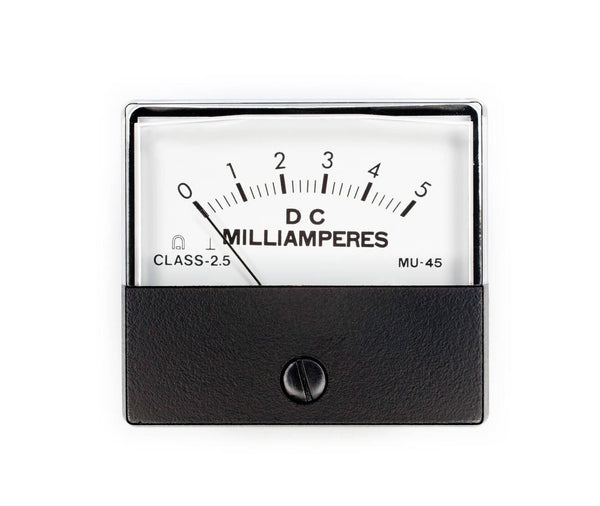mA to Watts Conversion (Simple Guide for Solar Conversions) - ShopSolar.com

# mA to Watts Conversion

One of the smartest energy decisions you can make is to switch to solar power. There are many benefits connected to solar energy from reducing your monthly utility bills to living a green lifestyle.

Deciding to switch is simple, but for solar novices, comprehending precise solar conversions can be a bit intimidating, especially when math isn’t your strong suit.

Many solar manuals tend to overcomplicate simple conversions such as milliamps (mA) to watts, which only causes more confusion among solar owners. However, it doesn’t have to be that difficult.

Fortunately, we'll demonstrate how simple it is to do the milliamp-to-watt conversion without having to consult a confusing manual. With our guide, you will learn everything you need to know about solar conversions and the simplicity involved in these calculations.

Electric current is very important in solar panel systems and getting the correct conversion is imperative to running a successful solar system and preventing damage. Using our household watt calculator will make you an expert in solar conversions.

So, sit back as begin our exploration of clean, renewable solar energy.

## What Is a Milliamp?Before we can get into the nitty-gritty of the conversions, it would be a good idea to give you a little insight into what a milliamp is.

A milliamp, abbreviated to mA, is a unit of measurement for the rate of electron flow as well as the amount of electric current flowing through an electrical conductor.

A milliamp is equal to one-thousandth of an ampere, which means that one milliamp is equal to 0.001 amperes.

Now that we have the definitions down, let us get into the simple math involved in the milliamp-to-watt conversion.

### The Conversion

So, we know that one milliamp is equal to 0.001 amperes. This means that 1,000 milliamps are similar to 1 amp. Interestingly, 1 milliamp is also equal to 0.001 watts; if we have this number, we can convert any milliamp amount.

To get to the wattage from milliamps all you need to do is multiply the milliamp number by 0.001. Let’s put it into perspective with a real-life scenario.Let’s say you want to work out the wattage of a milliamp reading of 5 mA. All you would need to do is take the 5 milliamps and multiply it by 0.001. This would give you a final watt reading of 0.005 watts.

As you can see, all you need to do is multiply the milliamp number by 0.001 each time and this gives you the respective wattage amounts.

For instance, if you want to know the wattage of your 1,000-milliamp solar system, again all you would need to do is multiply 1,000 by 0.001 to give you the watts. In this case, it would be 1 watt.

The final reading for watts is usually given in watts/volts which is the same thing, and it uses the same conversion rules.

There’s another simple formula you can use to work out watts and it is the reverse of the one listed above.

W = milliamps / 1,000, where W is equal to the watts.

This also gives you the wattage of any milliamp amount.

If we take the same example above of 1,000 milliamps, then all you would do is take the 1000 and divide it by 1,000. This gives you a watt value of 1.

Additionally, you can use the above formula to convert volts to watts by simply rearranging the formula.

## Conclusion

Working out solar electrical current conversions can initially be difficult, especially when you aren’t taken through the steps as a solar newbie. Thankfully, with this easy-to-follow guide, you will be able to convert any milliamp amount to its respective wattage.If you need further assistance in working out an electrical current conversion, then you can always visit Shop Solar Kits. We have excellent customer service and can lend a helping hand in all your solar energy queries from system setups involving solar panel inverters to general solar advice.

There are loads of other conversions and calculations that are a little more complex such as PV watt calculators, AC watt calculators, and mAh watt hours calculators.

The solar world is booming and it's time to get in on the energy trend taking the world by storm. Let’s live greener lives together and make the world a cleaner place by going solar.

Did You Find Our Blog Helpful? Then Consider Checking: